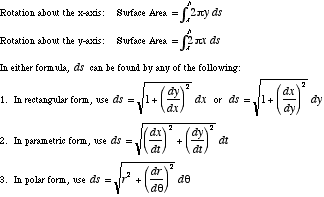index: click on a letter A B C D E F G H I J K L M N O P Q R S T U V W X Y Z A to Z index index: subject areas numbers & symbols sets, logic, proofs geometry algebra trigonometry advanced algebra & pre-calculus calculus advanced topics probability & statistics real world applications multimedia entrieswww.mathwords.com about mathwords website feedback

 Surface Area of a Surface of Revolution The formulas below give the surface area of a surface of revolution. The axis of rotation must be either the x-axis or the y-axis. The curve being rotated can be defined using rectangular, polar, or parametric equations.See also